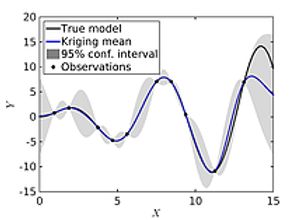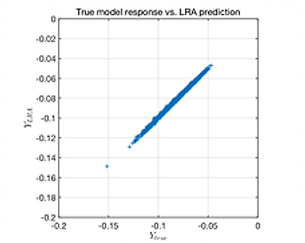###### Features at-a-glance

• Bayesian inversion

• UQLink: universal connection to third-party software

• Support vector machines for classification and regression

• Seamless connection with MATLAB-based models

• (Sparse) polynomial chaos expansions

• Advanced Kriging (Gaussian process modeling)

• Polynomial Chaos-Kriging (PC-Kriging)

• Canonical low-rank tensor approximations

• Local and global sensitivity analysis

• Reliability analysis (rare event estimation)

• UQLib: an open-source numerical library of UQLab

## NEW!

Bayesian inversion

UQLink allows the seamless connection of third-party software to UQLab using universal "wrapping" of external codes through templates and a mark-up system.

• Based on automated text input file generation

• Available for all platforms (Windows, Linux, macOS)

• Examples with simple C/C++ code and commercial finite element softwareto the examples

UQLink allows the seamless connection of third-party software to UQLab using universal "wrapping" of external codes through templates and a mark-up system.

• Based on automated text input file generation

• Available for all platforms (Windows, Linux, macOS)

• Examples with simple C/C++ code and commercial finite element softwareSupport vector machines

Support vector machines (SVM) come from machine learning and allow one to build predictive models from data. In the context of uncertainty quantification, SVM for regression (SVR) can be used as surrogate models of complex simulators using designs of computer experiments. SVM for classification (SVC) can be used in the context of reliability analysis.

• L1-SVR and L2-SVR formulation for regression

• Soft-margin classification

• Anisotropic and user-defined kernels

• Leave-one-out error and span approximations

• Multiple optimization algorithms (grid search, BFGS, cross-entropy, CMA-ES, etc.)In many uncertainty quantification problems, the sources of uncertainty are represented by complex probabilistic models (random vectors). UQLab offers a powerful and extendible set of tools to represent and sample from complex multivariate distributions.

• Extensive library of marginal distributions

• Modelling dependence with Gaussian copula

• Advanced sampling strategies (space-filling), including Monte-Carlo sampling, optimized latin hypercube sampling (LHS), low-discrepancy series (Sobol' and Halton sequences)

• Sampling enrichment (nested LHS)

• Support for custom-defined and bounded marginals

• Isoprobabilistic transform facilitiesEasy plug-in of MATLAB-based models

Uncertainty quantification aims at predicting the impact of input parameters uncertainty onto the predictions of a computational model. UQLab offers a simple infrastructure to handle analytical models and MATLAB-based computational models (solvers).

• Built-in support for functions defined as strings, function handles and m-files

• Intuitive integration of more complex codesto the examples
Polynomial chaos expansions

Polynomial Chaos Expansions (PCE) are a metamodeling tool that enables the fast construction of surrogate models, which can be efficiently used for moment- and sensitivity analysis.

• Full  and sparse polynomial chaos expansions

• Advanced truncation strategies (hyperbolic norms, max interaction, custom basis specification)

• Ordinary least squares, Least Angle Regression (LARS) and Orthogonal Matching Pursuit regression algorithms

• Degree-adaptive sparse polynomial chaos expansions

• Polynomials orthogonal to arbitrary distributions (via Stieltjes construction)to the examples
Kriging (Gaussian process modeling)

Gaussian process modeling is a flexible and robust technique to build fast surrogate models based on small experimental designs.

• Simple, ordinary and universal Kriging

• Highly customizable trend and correlation functions

• Maximum-likelihood- and cross-validation-based hyperparameter estimation

• Gradient-based, global and hybrid optimization methodsPolynomial chaos-Kriging (PC-Kriging)

Polynomial Chaos-Kriging (PCK) associates the global approximation behaviour of polynomial chaos expansions and the local accuracy of Kriging to provide a highly accurate surrogate model at low computational costs.

• Support for sequential and optimal construction of PC-Kriging

• Full control on both levels of approximation: polynomial chaos expansions and Kriging directly use the corresponding dedicated UQLab modules

• Support for sparse, adaptive and arbitrary polynomial chaos expansions

• Gradient-based, global and hybrid optimization methods for KrigingCanonical low-rank tensor polynomial approximations

Canonical low-rank approximations (LRA) are a powerful alternative to polynomial chaos expansions that are particularly effective in high dimension.

• Low-rank basis construction based on orthonormal polynomials

• Adaptive identification of maximum rank and polynomial degree via cross-validation

• Alternate least-square calculation of basis elements and coefficients

• Polynomials orthogonal to arbitrary distributions (via Stieltjes construction)Sensitivity analysis

The sensitivity analysis module contains screening- and global sensitivity analysis methods, which quantitatively measure the importance of each input parameter.

• Screening methods (Morris' elementary effects, Cotter indices)

• Linear measures: perturbation method (Taylor series expansion), standard regression coefficients, input/output correlation and their rank-based versions

• Sobol' indices computed by Monte Carlo simulation or analytically (from polynomial-chaos expansions and low-rank tensor approximations)Reliability analysis (rare event estimation)

When the performance of a system is affected by uncertainties on its characteristics or its environment, reliability can be assessed by computing probabilities of failure.

• FORM/SORM approximation methods

• Sampling methods (Monte Carlo, importance sampling, subset simulation)

• Kriging-based adaptive methods (AK-MCS, APCK-MCS)UQLib

UQLink allows the seamless connection of third-party software to UQLab using universal "wrapping" of external codes through templates and a mark-up system.

• Optimization (grid search, cross entropy, covariance matrix adaptation - evolution strategy, etc.)

• Kernel (stationary and non-stationary)

• Input/output processing (subsampling)